# Multivariate Probability Distributions Multivariate Random Variables In many

• Slides: 16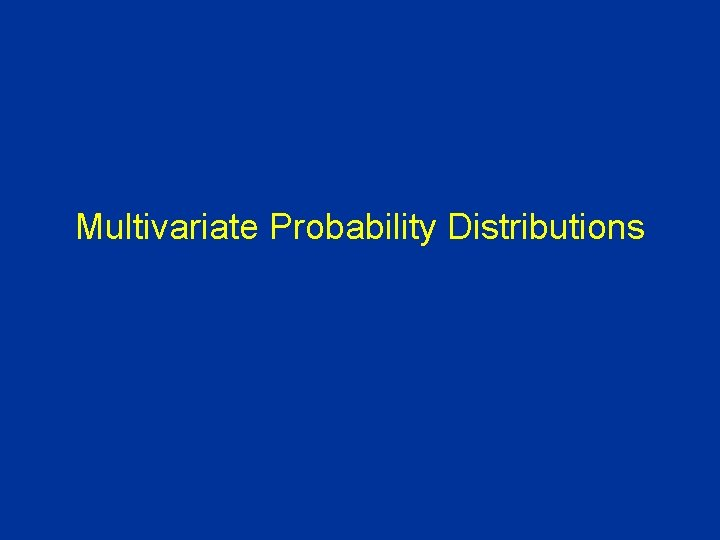Multivariate Probability DistributionsMultivariate Random Variables • In many settings, we are interested in 2 or more characteristics observed in experiments • Often used to study the relationship among characteristics and the prediction of one based on the other(s) • Three types of distributions: – Joint: Distribution of outcomes across all combinations of variables levels – Marginal: Distribution of outcomes for a single variable – Conditional: Distribution of outcomes for a single variable, given the level(s) of the other variable(s)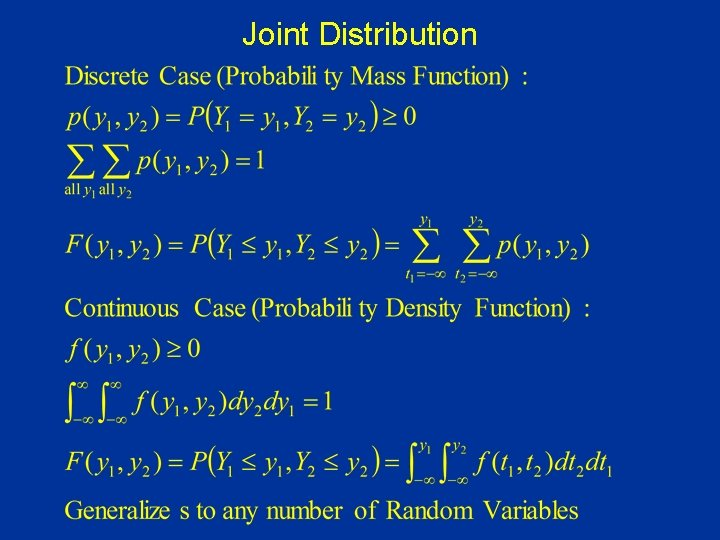Joint DistributionMarginal Distributions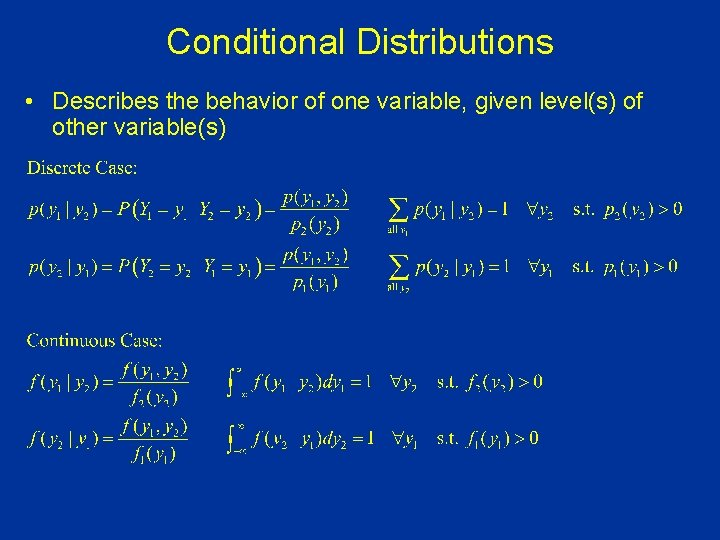Conditional Distributions • Describes the behavior of one variable, given level(s) of other variable(s)Expectations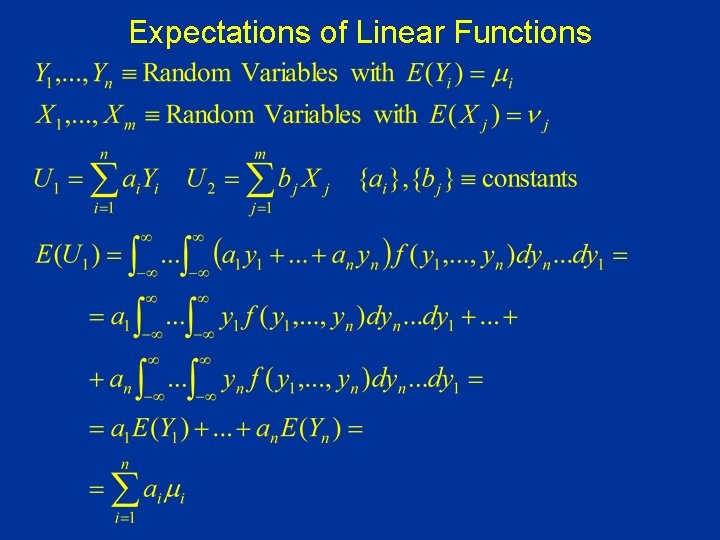Expectations of Linear FunctionsVariances of Linear FunctionsCovariance of Two Linear FunctionsMultinomial Distribution • Extension of Binomial Distribution to experiments where each trial can end in exactly one of k categories • n independent trials • Probability a trial results in category i is pi • Yi is the number of trials resulting in category I • p 1+…+pk = 1 • Y 1+…+Yk = nMultinomial DistributionMultinomial Distribution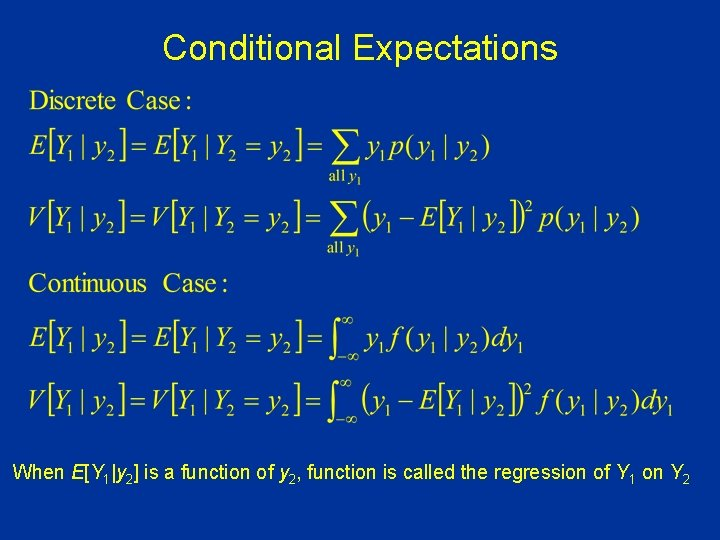Conditional Expectations When E[Y 1|y 2] is a function of y 2, function is called the regression of Y 1 on Y 2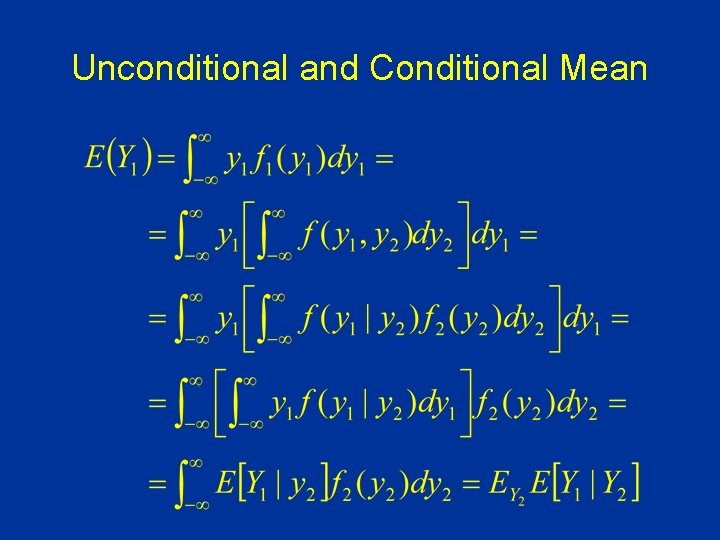Unconditional and Conditional Mean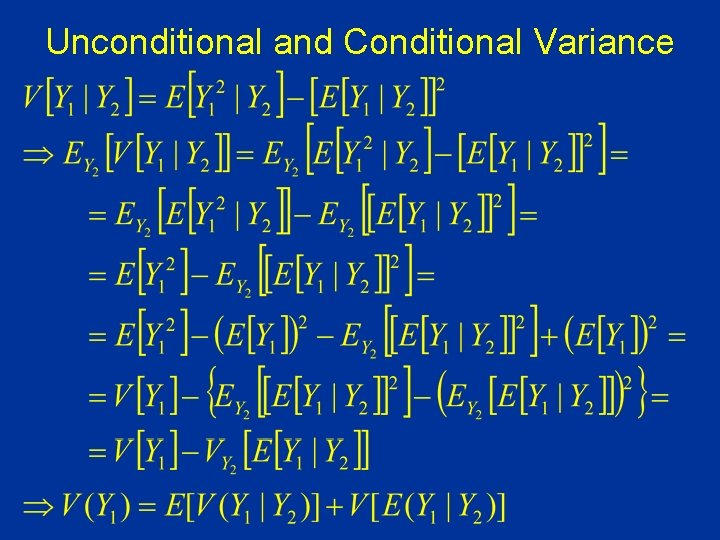Unconditional and Conditional Variance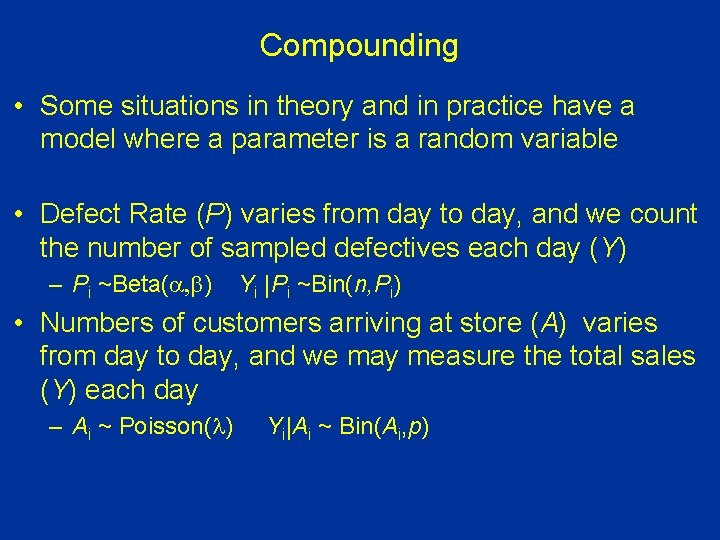Compounding • Some situations in theory and in practice have a model where a parameter is a random variable • Defect Rate (P) varies from day to day, and we count the number of sampled defectives each day (Y) – Pi ~Beta(a, b) Yi |Pi ~Bin(n, Pi) • Numbers of customers arriving at store (A) varies from day to day, and we may measure the total sales (Y) each day – Ai ~ Poisson(l) Yi|Ai ~ Bin(Ai, p)